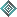Deja Vu, It's Algebra 2!Lesson 1: The Search for x - What is Algebra? (Notes, Key)Lesson 2: Notation, Functions, Domain & Range (Notes, Key)Lesson 3: Solving Linear Equations (Notes, Key)Lesson 4: Linear Functions - Graphing & Writing Equations (Notes, Key)Lesson 5: Absolute Value Equations, Inequalities, & Functions (Notes, Key)Lesson 6: Systems of Linear Equations (Notes, Key)Lesson 7: Linear Systems in 3-D (Notes, Key)Lesson 8: Introduction to Matrices (Notes, Key)Lesson 9: Matrix Multiplication (Notes, Key)Lesson 10: Determinants & Matrix Multiplication (Notes, Key)Lesson 11: Quadratic Functions - Graphs & Properties (Notes, Key)Lesson 12: Quadratic Functions - Reflections & Dilations, Roots, Max & Mins (Notes, Key)Lesson 13: Complex Numbers and Imaginary Roots (Notes, Key)Lesson 14: Polynomials - Addition, Subtraction, & Multiplication (Notes, Key)Lesson 15: Polynomials - Factoring & Synthetic Division (Notes, Key)Lesson 16: Polynomials - Finding Complex and Irrational Roots (Notes, Key)Lesson 17: Exponential Functions (Notes, Key)Lesson 18: Inverse & Logarithmic Functions (Notes, Key)Lesson 19: Properties of Logarithms (Notes, Key)Lesson 20: Exponential & Log Equations (Notes, Key)Lesson 21: Rational Expressions - Operations & Equations (Notes, Key)Lesson 22: Rational Expressions - Addition, Subtraction, & Complex Fractions (Notes, Key)Lesson 23: Rational Functions (Notes, Key)Lesson 24: Radical Functions, Expressions, & Operations (Notes, Key)Lesson 25: Representing Functions & Interpreting Graphs (Notes, Key)Lesson 26: Piecewise Functions (Notes, Key)Lesson 27: Building Functions from Functions (Notes, Key)Lesson 28: Conic Sections - Intro & Circles (Notes, Key)Lesson 29: Conic Sections - Ellipses (Notes, Key)Lesson 30: Conic Sections - Hyperbolas (Notes, Key)Lesson 31: Conic Sections - Parabolas (Notes, Key)Lesson 32: Permutations & Combinations (Notes, Key)Lesson 33: Abstract Algebra - A Look at Symmetry and Groups (Notes)